# RD Sharma Solutions Class 6 Maths Chapter 18 Basic Geometrical Tools

Read RD Sharma Solutions Class 6 Maths Chapter 18 Basic Geometrical Tools below, students should study RD Sharma class 6 Mathematics available on Studiestoday.com with solved questions and answers. These chapter wise answers for class 6 Mathematics have been prepared by teacher of Grade 6. These RD Sharma class 6 Solutions have been designed as per the latest NCERT syllabus for class 6 and if practiced thoroughly can help you to score good marks in standard 6 Mathematics class tests and examinations

Exercise 18.1

Question 1: Construct the following angles using set- squares:

(i) 45°

(ii) 90°

(iii) 60°

(iv) 105°

(v) 75°

(vi) 150°

Solution 1:1. Place set- square at 45°.
2. Draw two rays AB and AC along the edges from the vertex from the vertex of 45angle of the set- square.

So, the angle formed is 45° angle.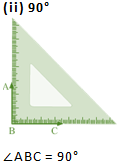1. Place set- square at 90°.
2. Draw two rays BC and BA along the edges from the vertex of 90° angle.

So, the angle formed is 90° angle.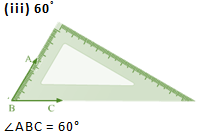1. Place set- square at 30°.
2. Draw the rays BA and BC along the edges from the vertex of 60°

So, the angle formed is 60° angle.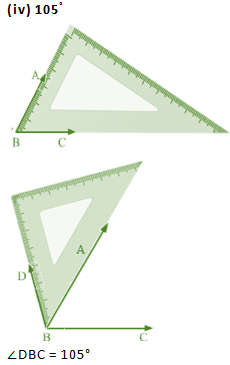1. Place 30° set –square and make an angle 60° by drawing the rays BA and BC.
2. Now place the vertex of 45°of the set –square on the ray BA as shown in figure and draw the ray BD.

So, the angle formed is 105° angle.1. Place 45° set –square and make an angle of 45° by drawing the rays BD and BC.
2. Now place the vertex of 30° of the set- square on the ray BD as shown in the figure and draw the ray BA.

So, the angle formed is 75° angle.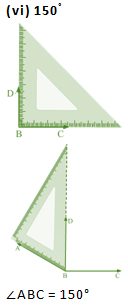1. Place the vertex of 45° of the set – square and make angle of 90o by drawing the rays BD and BC.
2. Now, place the vertex of 30°of the set –square on the ray BS as shown in the figure and draw the ray BA

So, the angle formed is 105° angle.

Question 2: Given a line BC and a point A on it, construct a ray AD using set – squares so that ∠DAC is

(i) 30°

(ii) 150°

Solution 2:

(i)  30° :

1. Make a line BC and take a point A on it.
2. Place 30° set –square on the line BC such that its vertex of 30° angle lies on point A and one edge coincides with the ray AB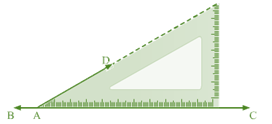(ii)     150°  :

1. Make a line BC and take a point A on it.

2. Place 30° set –square on the line BC such that its vertex of 30° angle lies on point A and one edge coincides with the ray AB.Therefore, ∠DAB = 30°

Angle on one side of the straight line is equal to 180o

Therefore, ∠DAB + ∠DAC = 180°

Therefore, ∠DAC = 150°

Exercise 18.2

Question 1: Mark the two points, A and B on a piece of paper and join them. Measure this length. For each of the following draw a line segment CD that is:

(i) Equal to the segment AB

(ii) Twice AB

(iii) Three times AB

(iv) Half AB

(v) Collinear with AB and is equal to it.

Solution 1:  Mark two points, A and B on a piece of paper and join them as follows:

For measure the length of AB, set the ruler with its edge along AB, such that the zero mark of the cm side of the ruler coincides with point A, according to the figure. Now, read the mark on the ruler, which corresponds to the point B. The length of the line segment AB. Here, AB = 5.6 cm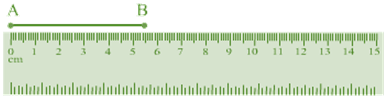(i)       Equal to the segment AB:

1. To draw the line segment CD equal to AB, take a divider and open it, from the first end-point A to second end- point B.

2. Then, lift the divider and place the end-points of both hands on the paper, where we have to draw CD, without disturbing its opening.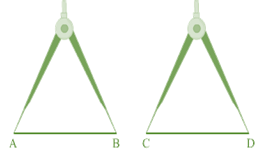(ii)   Twice AB :

1. To draw the line segment twice AB, draw a line l  and take a point C on the line.
2. Now, take divider and open it such that the end points of both its arms are at point A and point B.
3. Then, lift the divider and place one end-point at C and the other end-point on the line 1, without disturbing its opening.
4. Lift the divider and place point at E and the other end-point on the line 1, opposite C. Name this point D.(iii)     Three times AB :

1. To draw the line segment three times A, we draw a line l and take a point C on it.
2. Now take a divider and open it, such that the end-points of both its arms at A and B.
3. Then, we lift the divider and place one end-point at C and the other end-point on the line 1, Let this point be E.
4. Again, lift the divider and place one end-pint at E and the other end-point on the line 1, opposite to C. Let this point be F.
5. Again, lift the divider and place one end-point at F and the other end-point on the line 1, opposite to C. Name this point D.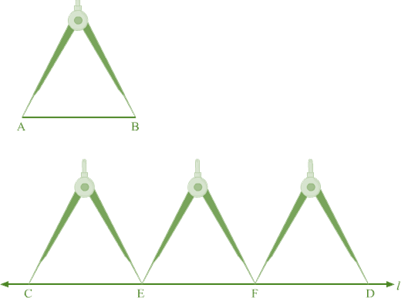(iv) Half AB :

1. To draw the line segment that is half AB, we draw a line l and take a point C on it.
2. Now, using a ruler, we measure the line segment AB and here, AB = 5.6 cm Half of AB = 5.62 = 2.8 cm
3. Now, we take a divider and open it so much that its end of one hand is at 0 and end of another hand is at 2.8 cm
4. Then, we lift the divider and place one end at C and the other end on the line l at point D.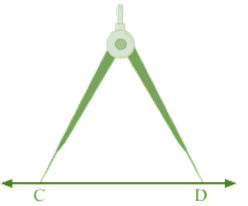(v)      Collinear with AB and is equal to it:

1. To draw a line segment CD collinear with AB and equal to AB, we take a ruler along AB and draw the line extended to AB.
2. We take a divider and open it such that the end-points of both its arms are at A and B.
3. Then, we lift the divider and place the end-points of both its hands on the extended line of AB and mark them as C and D.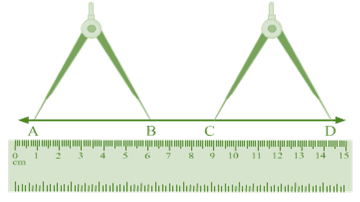Question 2:  The end – point P of a line – segment PQ is against 4 cm mark and the end – point Q is against the mark indicating 14.8 cm on a ruler. What is the length of the segment PQ?

Solution 2: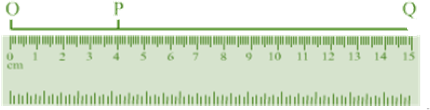Draw a line segment QP towards point 0 of the ruler and take a point 0 on the extended line QP corresponding to point 0 on the ruler.
According to the figure:
OP = 4 cm and OQ = 14.8 cm
Now, PQ = OQ – OP
= (14.8 – 4) cm
= 10.8 cm
Hence, OP is 10.8 cm.

Question 3: Draw a line segment CD. Produce it to CE such that CE = 3 CD

Solution 3:

1. Draw a line l and take two points C and D on the line.
2. Take a divider and open it from point C to end-Point D.
3. Then, we lift the divider and place its one end at D and other end on the line l opposite to C.
4. Let this point be A.
5. Lift the divider again and place its one end at A and other end on the line 1 opposite to C.
Name this point as E.
Here CD = DE = AE
Therefore, CE = CD + DE + AE
= CD + CD + CD (As, CD ± DE = AE)
or, CE = 3CD

Question 4:  If AB = 7.5 cm and CD = 2.5 cm, construct a segment whose length is equal to
(i) AB – CD
(ii) 2 AB
(iii) 3 CD
(iv) AB + CD
(v) 2 AB + 3 CD

Solution 4:   Given:

AB= 7.5 cm and CD = 2.5 cm

Draw AB and CD(i)       AB – CD:

1. Draw a line l and take a point E on the line.
2. Now, take a divider and open it so that the ends of both the arms are at A and B.
3. Then, uplift the divider and set its one end at E and another end (F) and on the line l.
4. Now, reset the divider in such a way that the end of its one hand is at C and the end of the other hand is at D.
5. Then, uplift the divider and place its one end at E and the other end (G) on the line l.
6. FG is required line segment, whose length is equal to (AB — CD)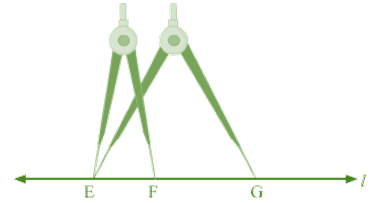(ii)                  2 AB :

1. Draw a line l and get a point E on it. Now a get a divider and open it such that the ends of both its arms are at A and B.
2. Then, uplift the divider and set its one end at E and another end (say F) on line l.
3. Again, lift the divider and place its one end F and another end on the line l, opposite to E.
4. Let this point be G. EG is required line segment, whose length is equal to 2 AB.(iii)       3 CD:

1. Draw a line l and take a point E on it. Now take a divider and open it such that the ends of both its arms are at C and D.
2. Then, we lift the divider and place its end at E on it and other end (F) on the line l.
3. Again, we lift the divider end (G) on the l opposite to C.
4. Again, lift the divider end (G) on the line l opposite to C.
5. Again, lift the divider and place its one end at G and another end (H) on the line l, opposite to E.
6. EH is required line segment, whose length is equal to 3 CD.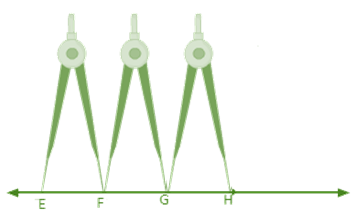(iv)       AB + CD :

1. We draw a line l and take a point E on it.
2. Now, take a divider and open it such that the ends of both its arms are A and B.
3. The, we lift the divider end (F) on the line l.
4. Now, reset the divider in such a way that the end of its one hand is at C and the end of the other hand is at D.
5. Then, we lift the divider and place its one end at F and another end (G) on the line l opposite to E as shown in the figure.
6. EG is required line segment, whose length is equal to (AB + CD)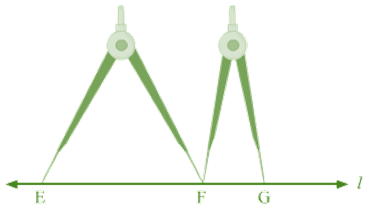(v)       2 AB + 3 CD :

1. Draw a line / and take point Eon it.
2. Now, take a divider and open it such that the ends of both its arms are at A and B.
3. Then, we lift the divider and place its one end at E and other end (say F) on the line 1, as shown in the figure.
4. Again, lift the divider and place its one end at F and another end (G) on the line 1, opposite to E.
5. Now, reset the divider in such a way that the ends of its one hand are at C and the end of other hand is at D.
6. Then, uplift the divider and place its one end at G and another end (say H) on the line 1, opposite to E.
7. Again, lift the divider and place its one end at H and other end (say I) on the line 1, opposite to E.
8. Again, lift the divider and place its one end at I and another end (say J) on the line 1, opposite to E.
9. EG is required line segment, whose length is equal to (2AB + 3CD).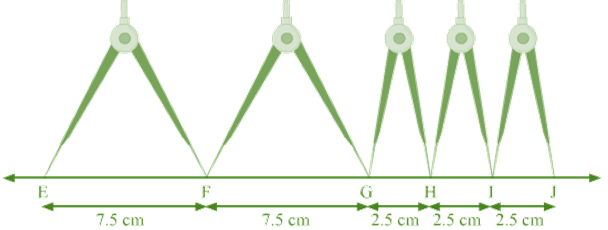Question 5:  Fill in the blanks:

(i) A part of a line with two end – points is called a _________.

(ii) Segment AB is _______ segment BA

(iii) The length of a line segment is the ___________ distance between two segments.

(iv) Two segments are congruent only if they have ________.

(v) Two segments of the same length are said to be _______.

Solution 5:

(i) A part of a line with two end – points is called a Line segment.

(ii) Segment AB is equal to segment BA.

(iii) The length of a line segment is the shortest distance between two segments.

(iv) Two segments are congruent only if they have equal length.

(v) Two segments of the same length are said to be congruent.

Question 6: Match the following statements: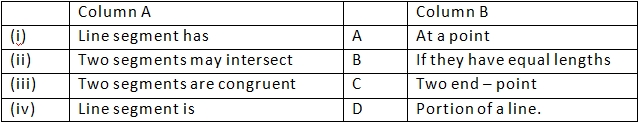Solution 6: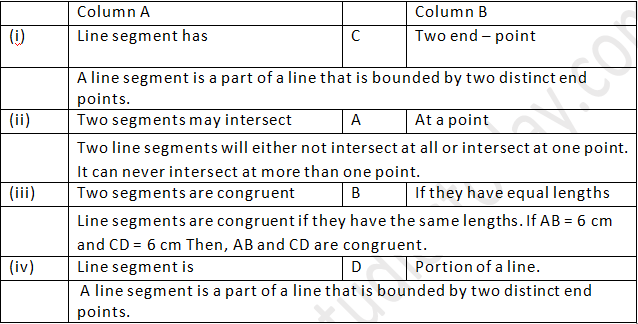Question 7: Tell which of the following statements are true (T) and false (F):

(i) The intersection of two segments may be segment.
(ii) Two segments may intersect at a point which is not any end point of either segments containing it.
(iii) Every ray is a segment
(iv) Every segment is a ray.

Solution 7:

(i) The intersection of two segments may be segment. False
(ii) Two segments may intersect at a point which is not any end point of either segments containing it. True
(iii) Every ray is a segment. True
(iv) Every segment is a ray. False

Question 8: What is the difference between a line, a line segment and a ray?:

Solution 8:A line can be drawn to infinity in both the directions. AB is a line

A line segment has both ends fixed. EF is a line segment. A ray has one end fixed and another end can be drawn to infinity. CD is a ray.

Question 9: How many rays are represented in fig 18.8? Name them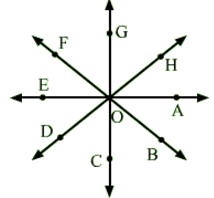Solution 9:    We know that a ray has fixed starting point and it can be drawn to infinity. If we take 0 as starting point, we will have a ray in every given direction.

So, our rays are, OA → , OB →, OC →, OD →, OE →, OF →, OG, OH→.

Thus, the number of rays in the figure is 8.